International
Tables for
Crystallography
Volume F
Crystallography of biological macromolecules
Edited by E. Arnold, D. M. Himmel and M. G. Rossmann

International Tables for Crystallography (2012). Vol. F, ch. 2.2, pp. 69-70

## Section 2.2.6. Quality indicators for density modification and phase improvement

H. M. Einspahra* and M. S. Weissb

aPO Box 6483, Lawrenceville, NJ 08648–0483, United States, and bHelmholtz-Zentrum Berlin für Materialien und Energie, Macromolecular Crystallography (HZB-MX), Albert-Einstein-Str. 15, D-12489 Berlin, Germany
Correspondence e-mail:  hmeinspahr@yahoo.com

### 2.2.6. Quality indicators for density modification and phase improvement

| top | pdf |

After determination of initial phases, a first electron-density map can be computed. It is expected that this map will contain significant errors and improbable features. Additional information, such as the flatness of the electron density in the solvent region or the similarity of electron-density regions of two or more identical molecules in the asymmetric unit, can be exploited to modify the electron density and hence improve the phases.

Figure of merit (f.o.m.), m. The figure of merit m [see equation (2.2.5.7)] is also used to judge the degree of improvement in the phase values. Again, in its most common use, it is defined as the weight applied to an individual structure-factor amplitude that, in conjunction with its best phase, gives rise, in a Fourier synthesis, to the electron-density map with the minimum level of noise. Typically, m is given as an average value over all reflections in the data set or in a given resolution shell.

Density-modification (DM) free R factor. The DM free R factor is defined in the same way as the refinement free R factor Rfree [see equation (2.2.8.1)and the following paragraph describing Rfree]. It describes the disagreement between the observed structure-factor amplitudes of a certain set of reflections and the calculated amplitudes after density modification. It is a weak indicator used in the program DM (Cowtan, 1999) and is helpful mainly in identifying the correct enantiomorph.

Density-modification (DM) real-space free residuals. The DM real-space free residuals are two numbers (real-space free residual for the solvent area and real-space free residual for the protein area) which are calculated by omitting two small spheres of protein and solvent from the density-modification process. The real-space free residual for the solvent area indicates how flat the solvent is in a non-flattened region of solvent and the real-space free residual for the protein area indicates how well the electron density in a non-histogram-matched region of protein obeys the expected histogram. The two residuals can also be combined into the combined real-space free residual by weighted summation, where the weight is defined by the solvent content. The DM real-space free residuals have some value in determining when to stop a density-modification calculation, once no more progress is being made, but limited value otherwise.

Contrast, c. The contrast c between the r.m.s. electron density in the solvent region and the r.m.s. electron density in the macromolecular region can serve as an indication of the presence of a clearly-defined solvent boundary in the electron-density map. It is defined as the standard deviation of the local r.m.s. electron-density values over the entire asymmetric unit (Terwilliger & Berendzen, 1999; Sheldrick, 2002; Terwilliger et al., 2009). The electron-density values are first squared (optionally after truncating very high and very low density values) and then smoothed using a moving local sphere typically with a radius of 6 Å. Local r.m.s. values are then calculated. The contrast c is now defined as the standard deviation σ of the local r.m.s. density values multiplied by a factor to normalize for the fraction of solvent sc in the crystal: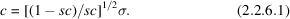Skewness of electron density, S. A high value for the skewness S of the electron density in an electron-density map indicates the presence of local electron-density maxima with high positive density values. The skewness is defined as the third moment of the electron density: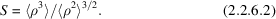In order to compute the mean values of ρ3 and ρ2, all density grid points in the asymmetric unit of the electron-density map are taken into account (Terwilliger et al., 2009).

Overlap of NCS-related density, ONCS. The presence of correlated electron density at noncrystallographic symmetry (NCS)-related regions in a map can be used as an indicator for the quality of the electron-density map (Cowtan & Main, 1998; Vellieux et al., 1995; Terwilliger et al., 2009). The overlap (ONCS) between density values at NCS-related locations is also often used to evaluate the presence of local symmetry or non-space-group symmetry: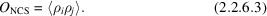ρi and ρj are the normalized electron-density values in the NCS-related regions in the asymmetric unit. The average is calculated over the whole region where NCS is present. This region may be defined as the region where overlap values are 0.3 or greater, or by a mask. If there are more than two NCS groups, the average is taken over all NCS pairs.

R factor, RDENMOD, and phase correlation, mDENMOD, from statistical density modification. The amplitudes and phases of structure factors calculated using statistical density modification can be compared with the observed amplitudes and experimental phases (Cowtan & Main, 1996; Terwilliger, 2001; Terwilliger et al., 2009). These comparisons yield an R value (RDENMOD) for the amplitudes and a mean cosine of the phase difference (mDENMOD) for the phases.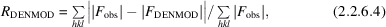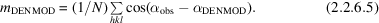Correlation coefficient CC of chain trace against native data. The quality of density modification in SHELXE (Sheldrick, 2002, 2010) can be assessed by computing a Pearson linear correlation coefficient [see equation (2.2.2.13)] of the calculated structure-factor amplitudes for a chain trace against the native structure-factor amplitudes. If the poly-Ala trace yields a CC value higher than 0.25 and if the mean chain length of the trace is >10, the solution is almost always correct. This criterion is currently implemented in the program ARCIMBOLDO (Rodriguez et al., 2009).

### References

Cowtan, K. (1999). Error estimation and bias correction in phase-improvement calculations. Acta Cryst. D55, 1555–1567.
Cowtan, K. & Main, P. (1998). Miscellaneous algorithms for density modification. Acta Cryst. D54, 487–493.
Cowtan, K. D. & Main, P. (1996). Phase combination and cross validation in iterated density-modification calculations. Acta Cryst. D52, 43–48.
Rodriguez, D. D., Grosse, C., Himmel, S., Gonzalez, C., de Ilarduya, I. M., Becker, S., Sheldrick, G. M. & Uson, I. (2009). Crystallographic ab initio protein structure solution below atomic resolution. Nat. Methods, 6, 651–653.
Sheldrick, G. M. (2002). Macromolecular phasing with SHELXE. Z. Kristallogr. 217, 644–650.
Sheldrick, G. M. (2010). Experimental phasing with SHELXC/D/E: combining chain tracing with density modification. Acta Cryst. D66, 479–485.
Terwilliger, T. C. (2001). Map-likelihood phasing. Acta Cryst. D57, 1763–1775.
Terwilliger, T. C., Adams, P. D., Read, R. J., McCoy, A. J., Moriarty, N. W., Grosse-Kunstleve, R. W., Afonine, P. V., Zwart, P. H. & Hung, L.-W. (2009). Decision-making in structure solution using Bayesian estimates of map quality: the PHENIX AutoSol wizard. Acta Cryst. D65, 582–601.
Terwilliger, T. C. & Berendzen, J. (1999). Discrimination of solvent from protein regions in native Fouriers as a means of evaluating heavy-atom solutions in the MIR and MAD methods. Acta Cryst. D55, 501–505.
Vellieux, F. M. D. A. P., Hunt, J. F., Roy, S. & Read, R. J. (1995). DEMON/ANGEL: a suite of programs to carry out density modification. J. Appl. Cryst. 28, 347–351.